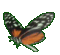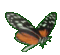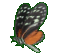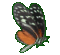Algebra Help - Formulas & Data Help - Game Tips:

- The formula, Distance = Speed x Time represents a large set of data in one simple equation D=ST.
An example of such data is D = 12 metres, S = 4 m/s and T = 3 seconds, since 12 = 4 times 3.

- If Speed is a constant S=4, the formula D=4T represents a set of number pairs having the form (T,D).
The formula D=4T represents a set of number pairs including the examples: (3,12) , (5,20) , (1,4) , etc.

- Similarly, Einsteins's formula, E=mc2 represents a relation between energy 'E' and mass 'm' .
When the speed of light 'c' is considered constant, Einstein's formula can be expressed as
E=(constant)m     or     E=(k)m .
Every mass 'm' at rest has an associated energy 'E' and can be represented as a data pair (m,E).

- Your Game Score is reduced by the number of butterfly hits.

- To slow the game speed repeat tap/click on the word Slider.
- To increase the game speed repeat tap/click on the word Math.
- Speed can also be adjusted with a keyboard's - and + keys.

- Refresh/Reload the web page to restart the game.